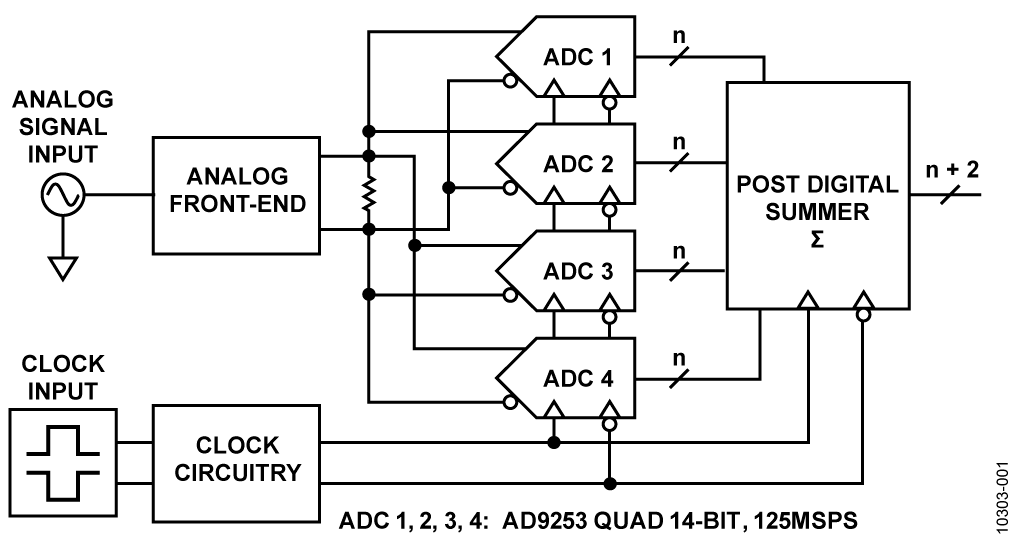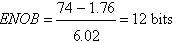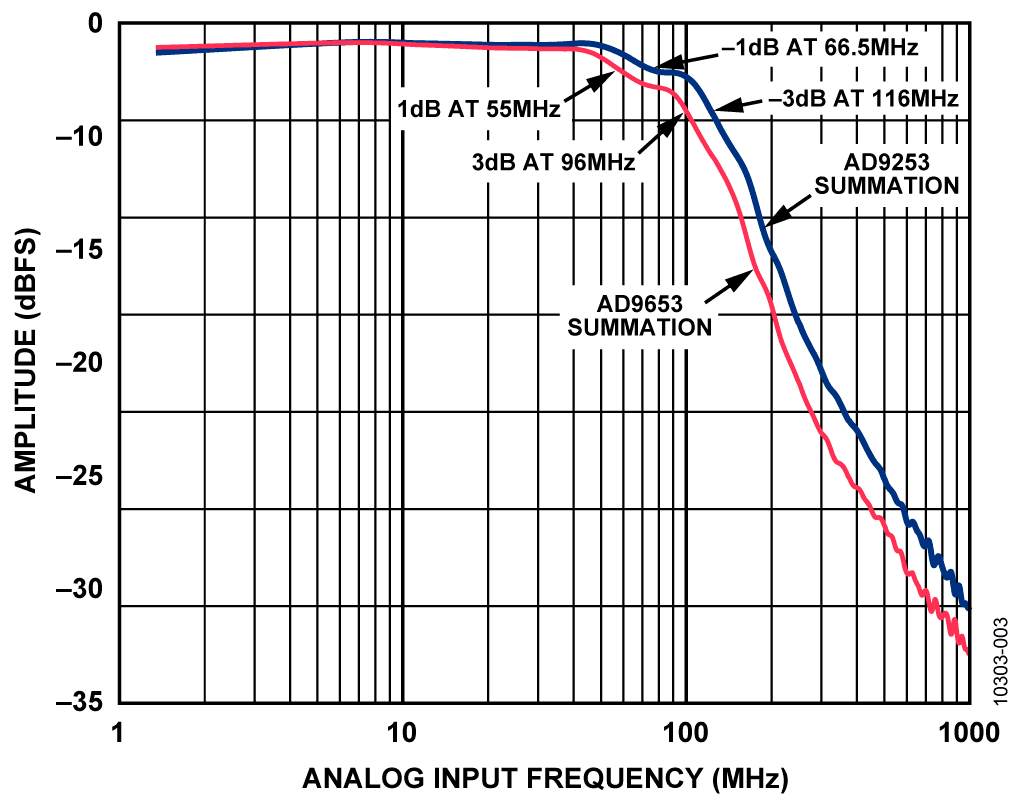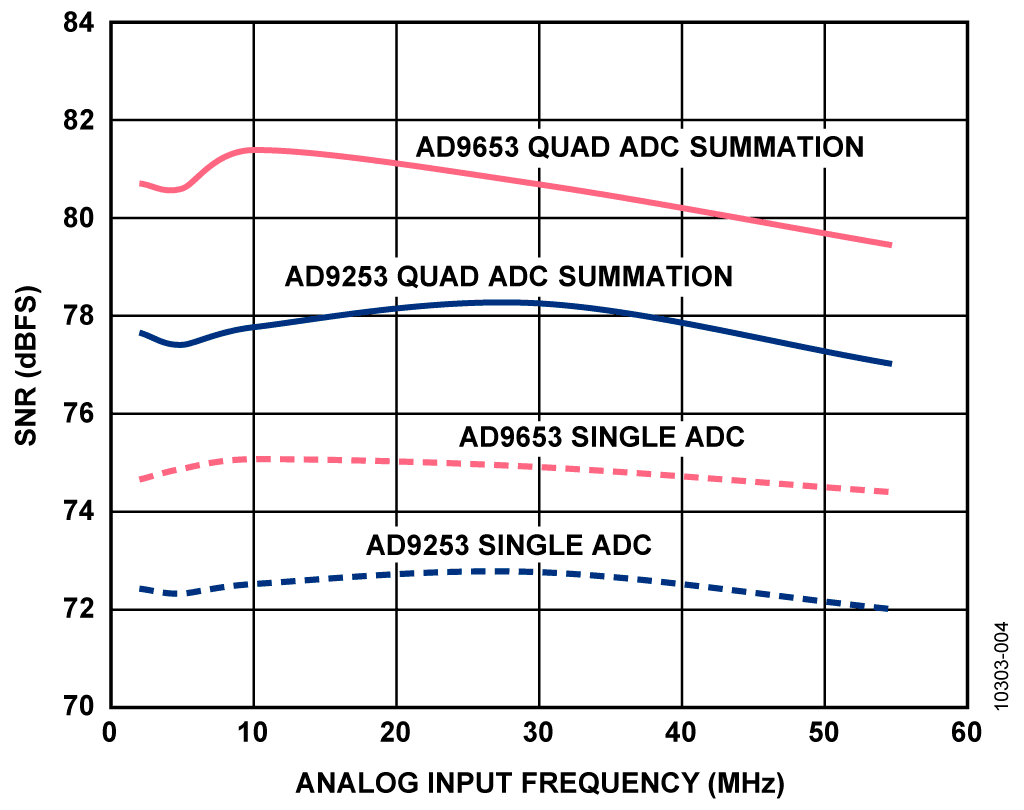Evaluation Hardware \$698.28•Overview

Design Resources

Design & Integration Files

• Schematic
• Bill of Materials
• Gerber Files
• Assembly Drawing

Evaluation Hardware

Part Numbers with "Z" indicate RoHS Compliance. Boards checked are needed to evaluate this circuit

• HSC-ADC-EVALCZ (\$698.28) Controller board needed to evaluate this circuit. Please see "Circuit Evaluation & Test" section for connection information.

Features & Benefits

• 78.5dBFS SNR
• Post digital summation enhancement

Circuit Function & Benefits

The circuit shown in Figure 1 is a simplified diagram of a 14-bit, 125 MSPS quad ADC system that uses post digital summation to increase the signal-to-noise ratio (SNR) from 74 dBFS for a single ADC to 78.5 dBFS for the quad ADC with summation. This technique is especially suitable for applications requiring high SNR such as ultrasound and radar, and makes use of modern high performance low power quad pipelined ADCs.

The circuit makes use of the fundamental principle that uncorrelated noise sources add on a root-sum-square (rss) basis, while signal voltages add on a linear basis.Figure 1. Basic Block Diagram of Summing Four ADC in Parallel to Achieve Higher SNR

Circuit Description

The input to each ADC consists of a signal term (VS) and a noise term (VN). Summing four noisy voltage sources results in a total voltage, VT, which is the linear sum of the four signal voltages plus the root-sum-square of the four noise voltages, for example.

Since VS1 = VS2 = VS3 = VS4, the signal has effectively been multiplied by four, while the converter noise—with equal rms values—has been multiplied by only two, thereby increasing the signal-to-noise ratio by a factor of two, or 6.02 dB. Thus, the 6.02 dB increase in SNR results from summing four like signals gives rise to one additional bit of effective resolution. Since SNR(dB) = 6.02N + 1.76 dB, where N is the number of bits,Table 1 shows the theoretical SNR that results from summing the outputs of multiple ADCs. From the standpoint of simplicity, summing four ADCs is an obvious choice. Larger numbers may also be of interest in critical cases, but that would depend on other system specifications (including cost) and the amount of board space available.

Table 1. Increase in SNR vs. Number of ADCs

 Number of ADCs Increase in SNR (dB) 2 3 4 6 8 9 16 12 32 15

The ideal SNR for a 14-bit ADC is (6.02 × 14) + 1.76 = 86.04 dB. The AD9253 data sheet specifies a typical SNR of 74 dB, however, yielding an ENOB of 12 bits.The circuit shown in Figure 1 has a passive receiver front end that combines four analog input channels together, based on the AD9253 14-bit, 125 MSPS quad channel, analog-to-digital converter.

The circuit accepts a single-ended input and converts it to differential using two wide bandwidth (3 GHz) M/A-COM ETC1-1-13 1:1 impedance ratio balun in a double balanced configuration as shown in Figure 2.Figure 2. Input Analog Summation Network

All four ADC inputs are connected together at the secondary of the balun configuration. No gain is applied in the circuit, and there is simple filtering at each analog input pair to reduce the amount of residual kickback that could potentially feedback into adjacent ADC channels.

The fully differential architecture through the ADC provides good high frequency common-mode rejection so that uncorrelated noise sources are minimized when summed yielding a perfor-mance of 78.5 dBFS SNR and 85dBc SFDR in the first Nyquist band (0 MHz to 62.5 MHz when sampling at 125 MSPS). The overall circuit has a bandwidth of 65 MHz with a pass band flatness of 1 dB.

To achieve best performance, a double-balanced balun approach was used to achieve the best even order spurious performance over frequency. Because the inputs of four ADCs were connected together, maintaining balance can be a challenge even at frequencies less than 100 MHz.

A 66 Ω differential termination was used to terminate the secondary of the balun configuration. The value of 66 Ω was chosen to help reduce the loss of the parallel combination of the four converter input impedances and minimize the loss seen from the secondary to the primary of the transformer, yielding an overall impedance of approximately 50 Ω, as seen from the primary.

Ferrite beads were employed in this design to help reduce the effects of parasitic capacitive loads from the board layout as well as the parallel combination of the four unbuffered ADC channels. The beads reduce the kickback from each of the ADC input channels thus preserving overall bandwidth.

The 10 Ω series resistors serve a dual purpose. First, they drive the ADC input filters (2 pF common-mode and 5 pF differential), second, they serve to reduce the amount of kickback that comes from each ADC. For more information on charge kickback and unbuffered ADC architectures, see Application Note AN-742.

The measured performance of the system is summarized in Table 2, where the −3 dB bandwidth is 67 MHz. The total insertion loss of the network is approximately 3 dB, therefore an input drive of +13 dBm is needed to provide a full-scale 2 V p-p differential signal to the input of the ADCs.

Table 2. Measured Performance of the Circuit

 Performance Specs at 2.0 V p-p FS Final Results Sample Frequency 125 MSPS Pass-Band Flatness (67 MHz) 3 dB SNRFS at 10 MHz 78.5 dBFS SFDR at 10 MHz 85 dBc H2/H3 at 10 MHz 85 dBc/90 dBc Input Impedance at 10 MHz 58 Ω Input Drive at 10 MHz +13.0 dBm

System PerformanceSNR was measured for both the single and quad versions of the AD9253 and AD9653 and is shown in Figure 4.Figure 4. SNR Performance vs. Frequency for Single and Quad Summation Configurations of the AD9253 and AD9653

Note that the use of the quad summation technique increases the SNR of the AD9253 14-bit ADC at 10 MHz by approximately 5 dB. The SNR of the AD9653 16-bit ADC is increased by approximately the same amount.

SFDR data was taken for the quad summation configuration of the AD9253 and AD9653, and it is shown in Figure 5.The input impedance of the circuit shown in Figure 1 and Figure 2 was measured using a network analyzer calibrated to 50 Ω over a 1 GHz band, as shown in Figure 6. The final network was found to have a VSWR of 1.2 or less over the desired band (1st Nyquist zone, dc to 62.5 MHz).Figure 6. Input Impedance of Complete Frontend Four-Channel Summation

Front End Interface Design Procedure

In this section, a general approach to the design of the passive front end ADC interface with filter is presented for the passive summation technique. To achieve optimum performance (bandwidth, SNR, and SFDR), there are certain design constraints placed on the general circuit by the front end and the ADC:

• Know and understand the key parameters in designing a front end. These include the following:

• Input impedance/VSWR or voltage standing wave ratio, is a unitless parameter that shows how much power is being reflected into the load over the bandwidth of interest. Input impedance of the network is the specified value of the load, usually 50 Ω.
• Passband flatness is usually defined as the amount of fluctuation ripple that can be tolerated within the specified bandwidth.
• Bandwidth is simply the range of the frequencies to be used in the system.
• Minimum SNR (signal-to-noise ratio) and SFDR (spurious free dynamic range)
• Input drive level is a function of the bandwidth, input impedance, and VSWR specifications. This sets the gain and amplitude required for a full-scale input signal at the converter. It is highly dependent on the front-end components chosen, for example, the transformer, amplifier, or anti-aliasing filter, and can be one of the most difficult parameters to achieve.

• The correct amount of series resistance between the ADC and the load presented by the filter must be determined. This is to prevent undesired peaking in the pass band and minimize the kickback from the individual ADC inputs. In most cases, the correct value must be determined empirically.
• The input impedance of the ADC may need to be lowered by shunting it with an external parallel resistor.
• The correct series resistance should be used to isolate the ADC from the filter. This series resistor also reduces peaking and is usually determined empirically.

The parameters in the interface circuit are very interactive; therefore, it is almost impossible to optimize the circuit for all key specifications (bandwidth, bandwidth flatness, SNR, SFDR, and gain).

In Figure 2, the pass-band peaking can be reduced as the value of the series resistance, RA, is increased. However, as the value of this resistance increases, there is more signal attenuation, and the input network must be driven with a larger signal to fill the parallel combination of all ADCs full-scale input range.

Balancing these trade-offs can be somewhat difficult. In this design, each parameter was given equal weight; therefore, the values chosen are representative of the interface performance for all the design characteristics. In some designs, different values may be chosen to optimize SFDR, SNR, or input drive level, depending on system requirements.

The SNR performance in this design is determined by several factors: the nature of the ADC architecture and the setting of the internal front-end buffer bias current in the AD9253 via the internal sample and hold mechanisms as well as the bandwidth required for the design. In this case, the entire first Nyquist was used.

Another trade-off that can be made in this particular design is the ADC full-scale setting. The full-scale ADC differential input voltage was set for 2 V p-p for the data obtained with this design, which optimizes SFDR. Changing the full-scale input range to lower than the maximum full scale of 2.0 V p-p yields a degradation in SNR performance.

Passive Component and PC Board Parasitic Considerations

The performance of this or any high speed circuit is highly dependent on proper PCB layout. This includes, but is not limited to, power supply bypassing, controlled impedance lines (where required), component placement, signal routing, and power and ground planes. See Tutorials MT-031 and MT-101 for more detailed information regarding PCB layout for high speed ADCs and amplifiers.

Use low parasitic surface-mount capacitors, inductors, and resistors for the passive components in the filter. The inductors chosen are from the Coilcraft 0603CS series. The surface mount capacitors used in the filter are 5%, C0G, 0402-type for stability and accuracy.

See the CN-0249 Design Support Package for complete documentation on the system.

Common Variations

For applications that require the same bandwidth, lower power and lower performance the AD9633 12-bit, 125 MSPS quad channel A/D converter can be used. For applications that require the same bandwidth, slightly higher power, and higher performance, the AD9653 16-bit, 125 MSPS quad channel A/D converter can be used. These devices are pin compatible with the others previously listed.

Circuit Evaluation & Test

This circuit uses a modified AD9253-125EBZ circuit board and the HSC-ADC-EVALCZ FPGA-based data capture board. The two boards have mating high speed connectors, allowing for the quick setup and evaluation of the circuit’s performance. The modified AD9253-125EBZ board contains the circuit evaluated as described in this note, and the HSC-ADC-EVALCZ data capture board is used in conjunction with Visual Analog evaluation software, as well as the SPI Controller software to properly control the ADC and capture the data. The CN-0249 Design Support package at http://www.analog.com/CN0249-DesignSupport contains the schematics, BOM, and layout for the board. Application Note AN-835 contains complete details on how to set up the hardware and software to run the tests described in this circuit note. The user guide, Evaluating the AD9653/ AD9253/ AD9633 Analog-to-Digital Converters describes the basic evaluation procedure for the AD9253.

Sample Products

Samples

 Product Description Available Product Models to Sample AD9253 Quad, 14-Bit, 80 MSPS/105 MSPS/125 MSPS Serial LVDS 1.8 V Analog-to-Digital Converter
Buy functionality exists in desktop site
Evaluation Boards Pricing displayed is based on 1-piece.
Pricing displayed is based on 1-piece. The USA list pricing shown is for budgetary use only, shown in United States dollars (FOB USA per unit), and is subject to change. International prices may vary due to local duties, taxes, fees and exchange rates.